# NCERT Solutions for Class 7 Maths Chapter 1 Integers

Class 7 Maths NCERT Solutions Chapter 1 – Integers comprises of the 4 Exercises,

This Chapter contains the Exercises relating to the following topics , which are discussed in Chapter 1 – Integers Class 7 NCERT book  : –

• 1.1 INTRODUCTION
• 1.2 RECALL
• 1.3 PROPERTIES OF ADDITION AND SUBTRACTION OF INTEGERS
• 1.3.2 Closure under Subtraction
• 1.3.3 Commutative Property
• 1.3.4 Associative Property
• 1.4 MULTIPLICATION OF INTEGERS
• 1.4.1 Multiplication of a Positive and a Negative Integer
• 1.4.2 Multiplication of two Negative Integers
• 1.4.3 Product of three or more Negative Integers
• 1.5 PROPERTIES OF MULTIPLICATION OF INTEGERS
• 1.5.1 Closure under Multiplication
• 1.5.2 Commutativity of Multiplication
• 1.5.3 Multiplication by Zero
• 1.5.4 Multiplicative Identity
• 1.5.5 Associativity for Multiplication
• 1.5.6 Distributive Property
• 1.5.7 Making Multiplication Easier
• 1.6 DIVISION OF INTEGERS
• 1.7 PROPERTIES OF DIVISION OF INTEGERS

### Class 7 Maths NCERT Solutions Chapter 1 – Exercise 1.1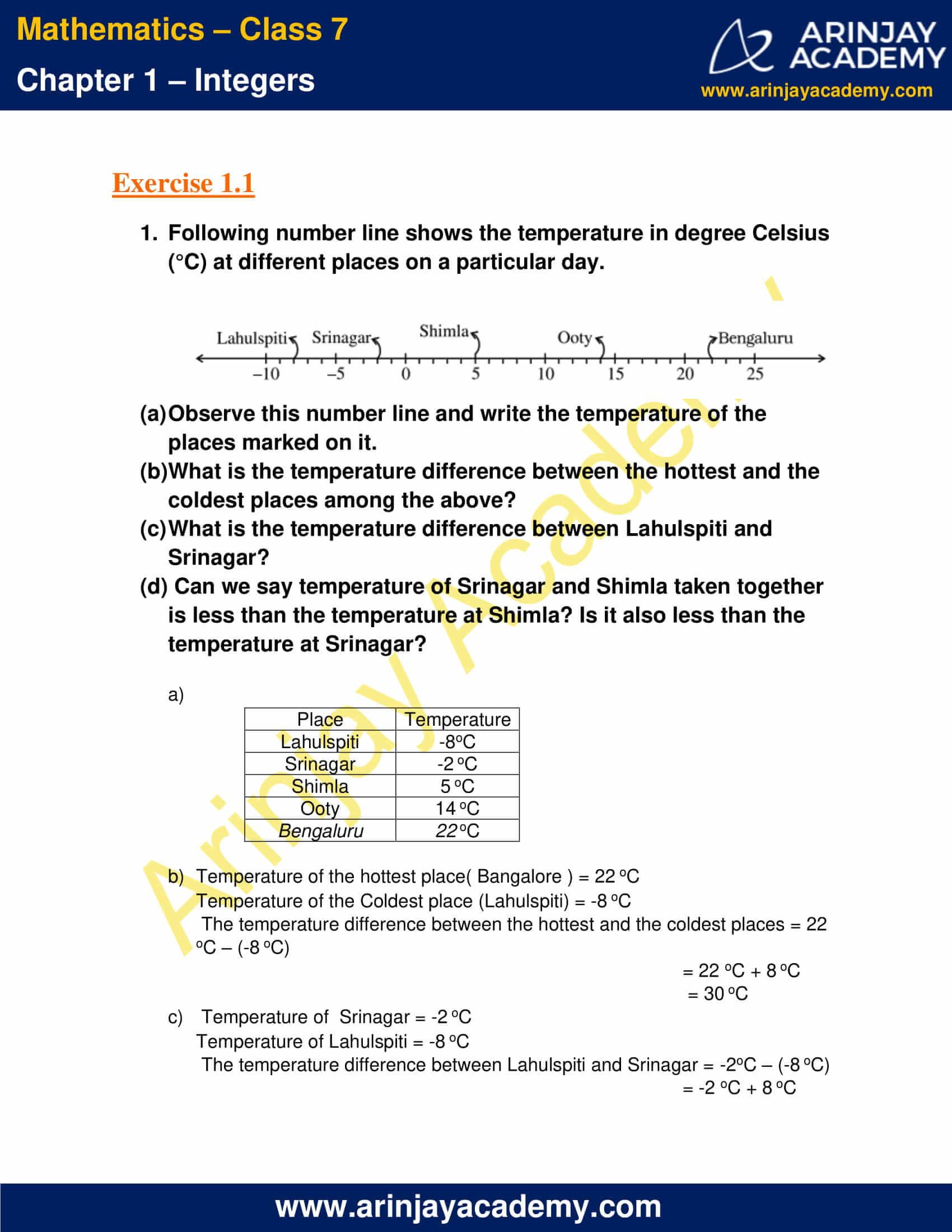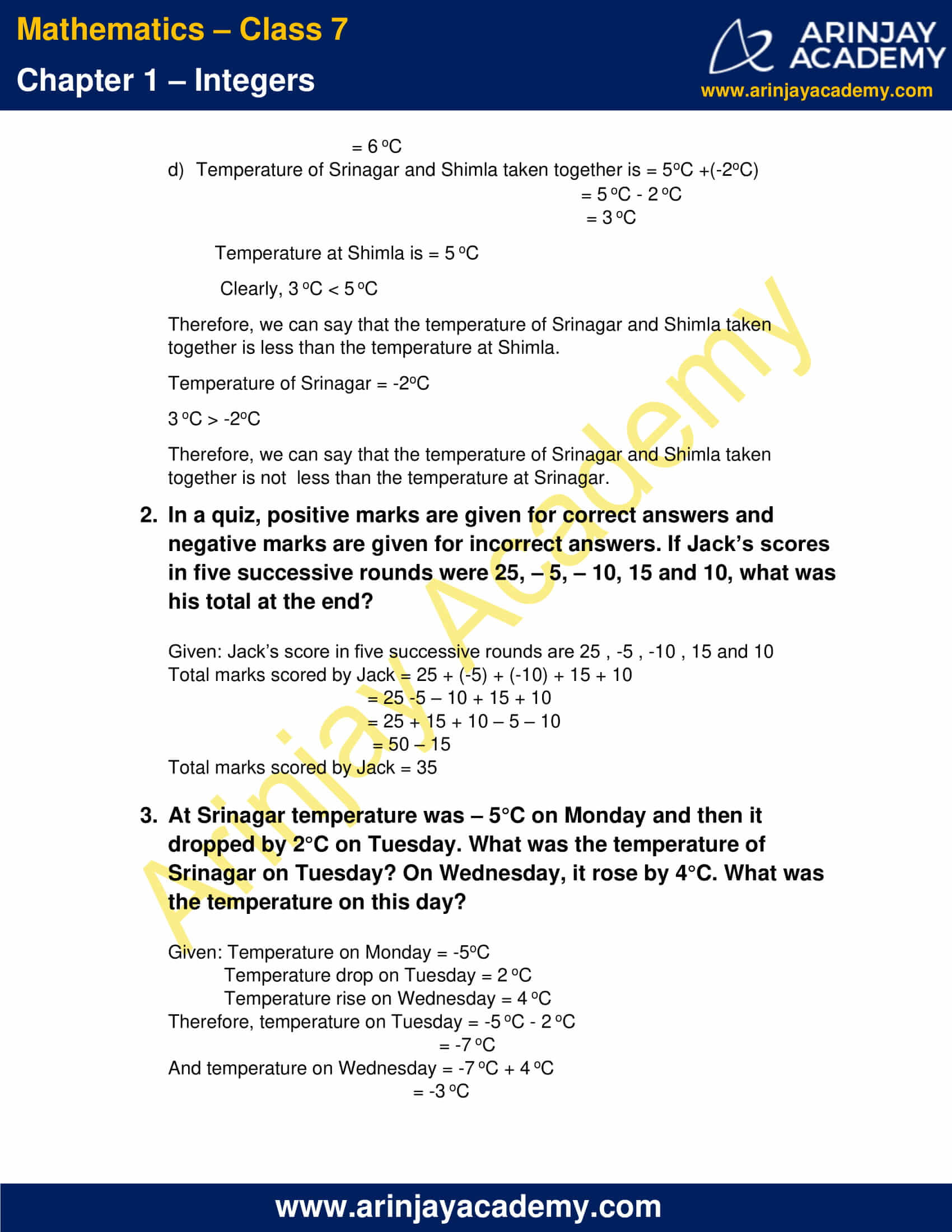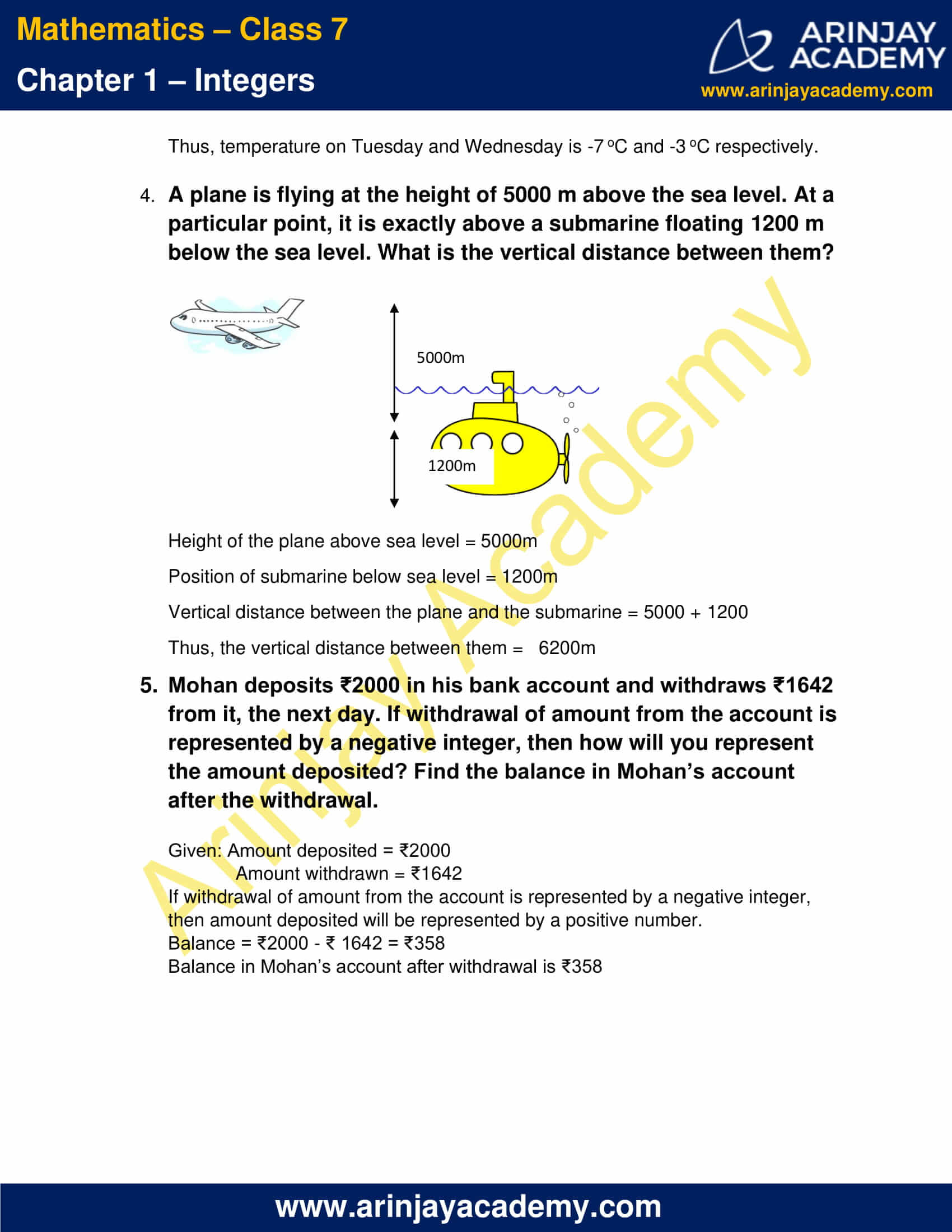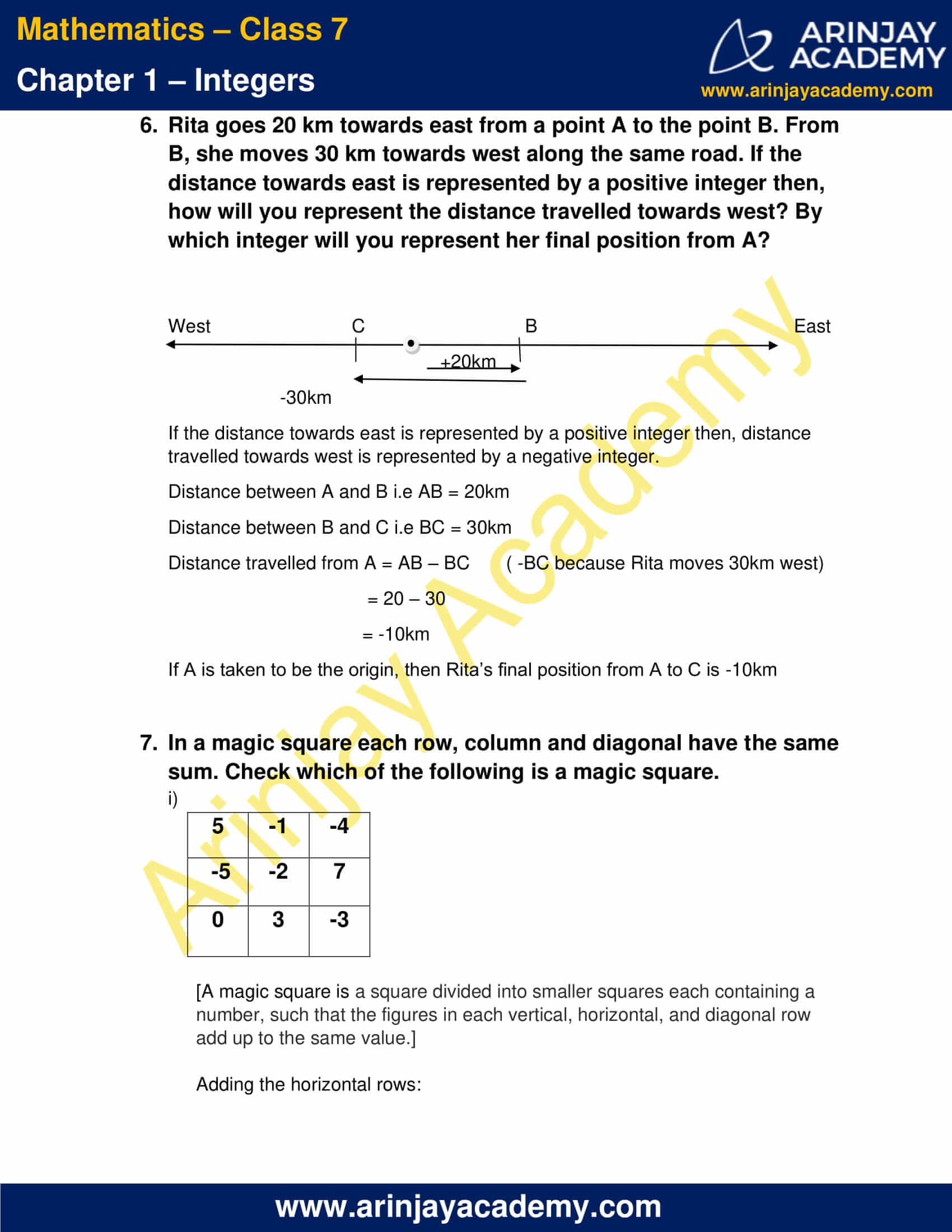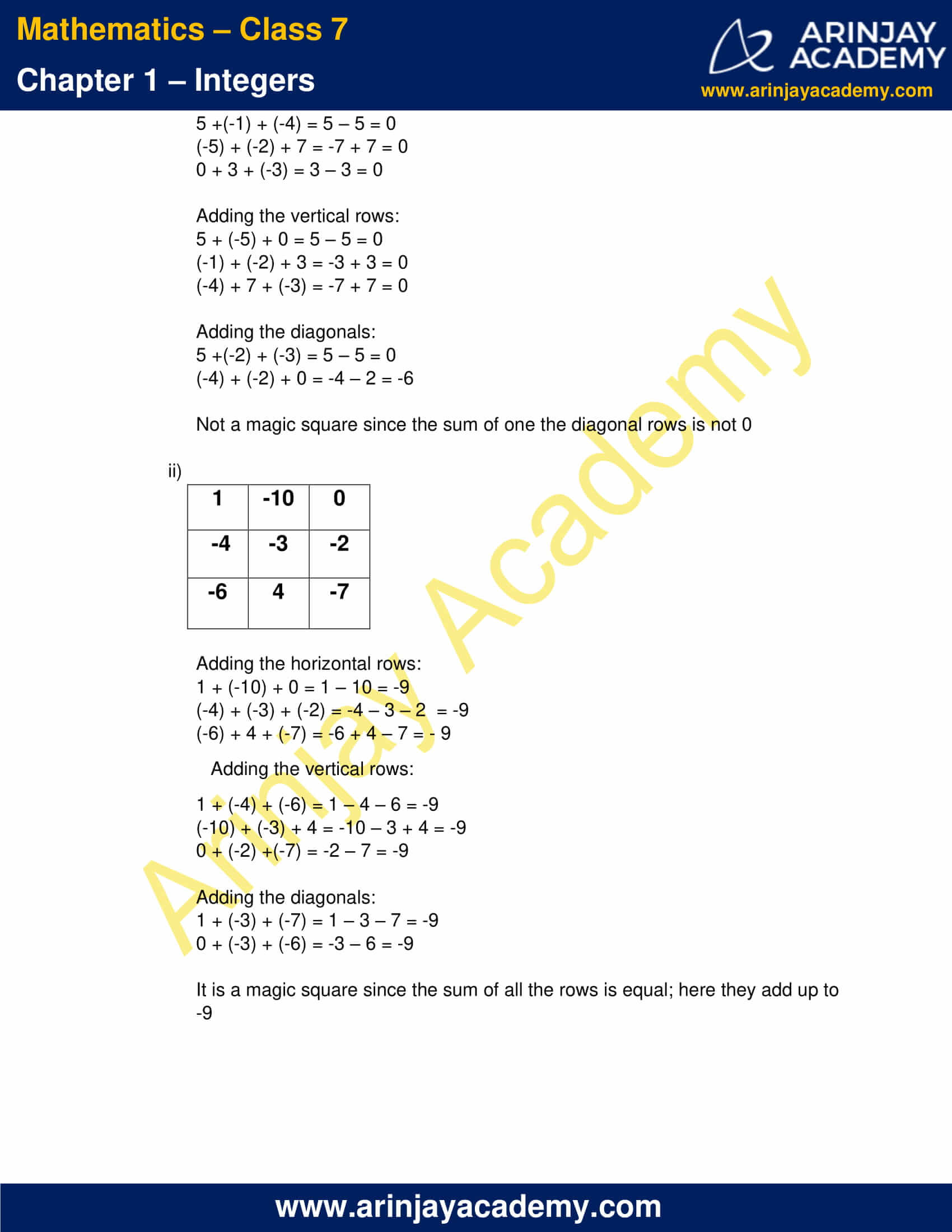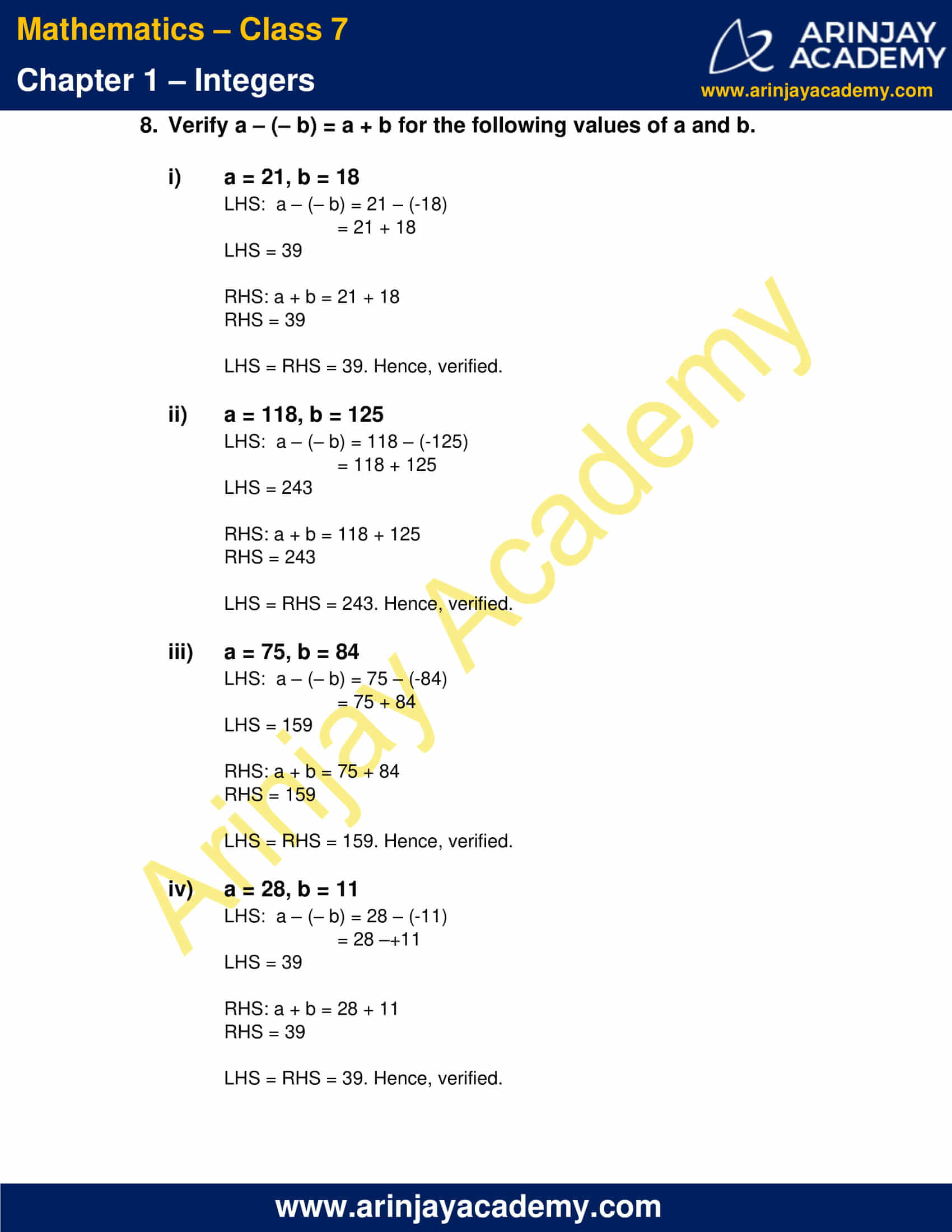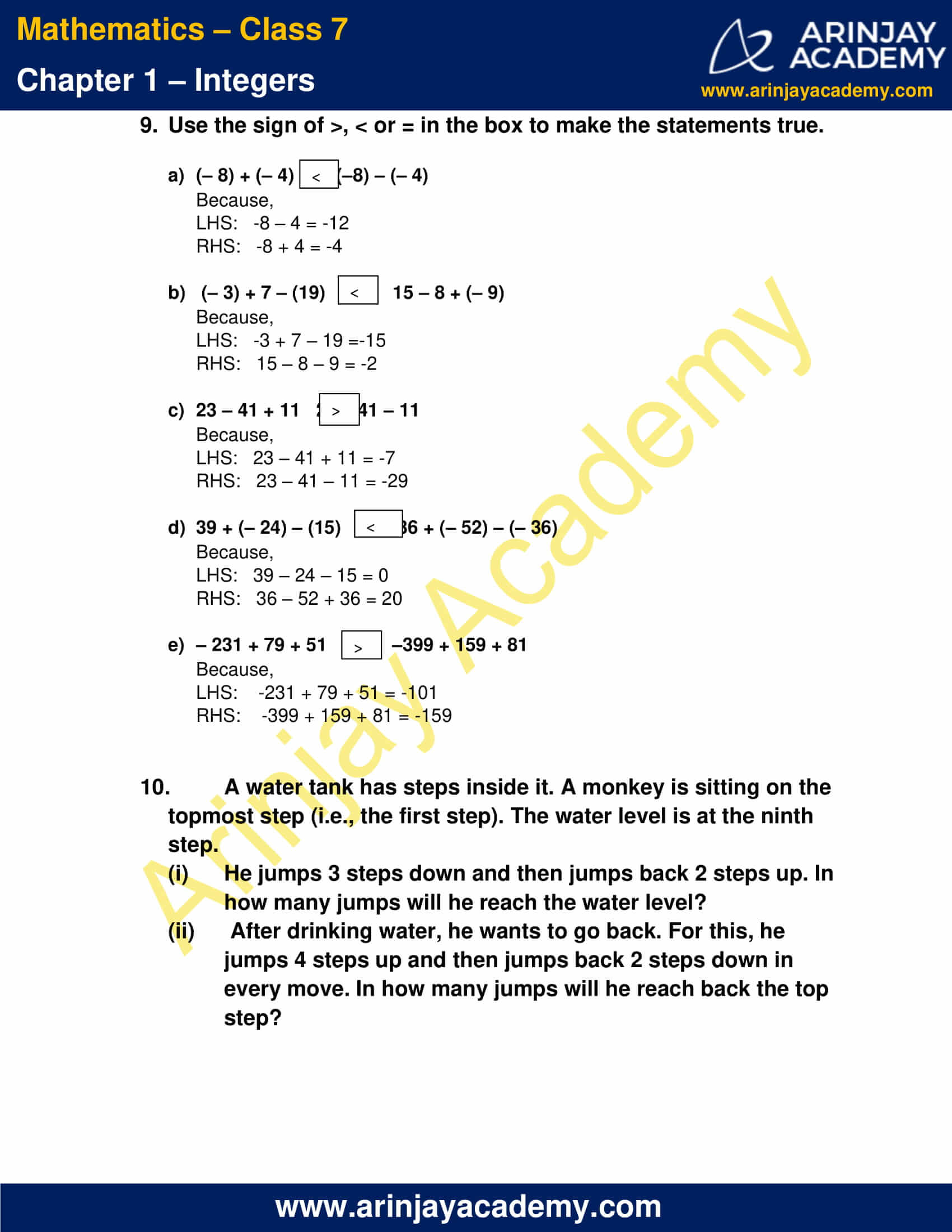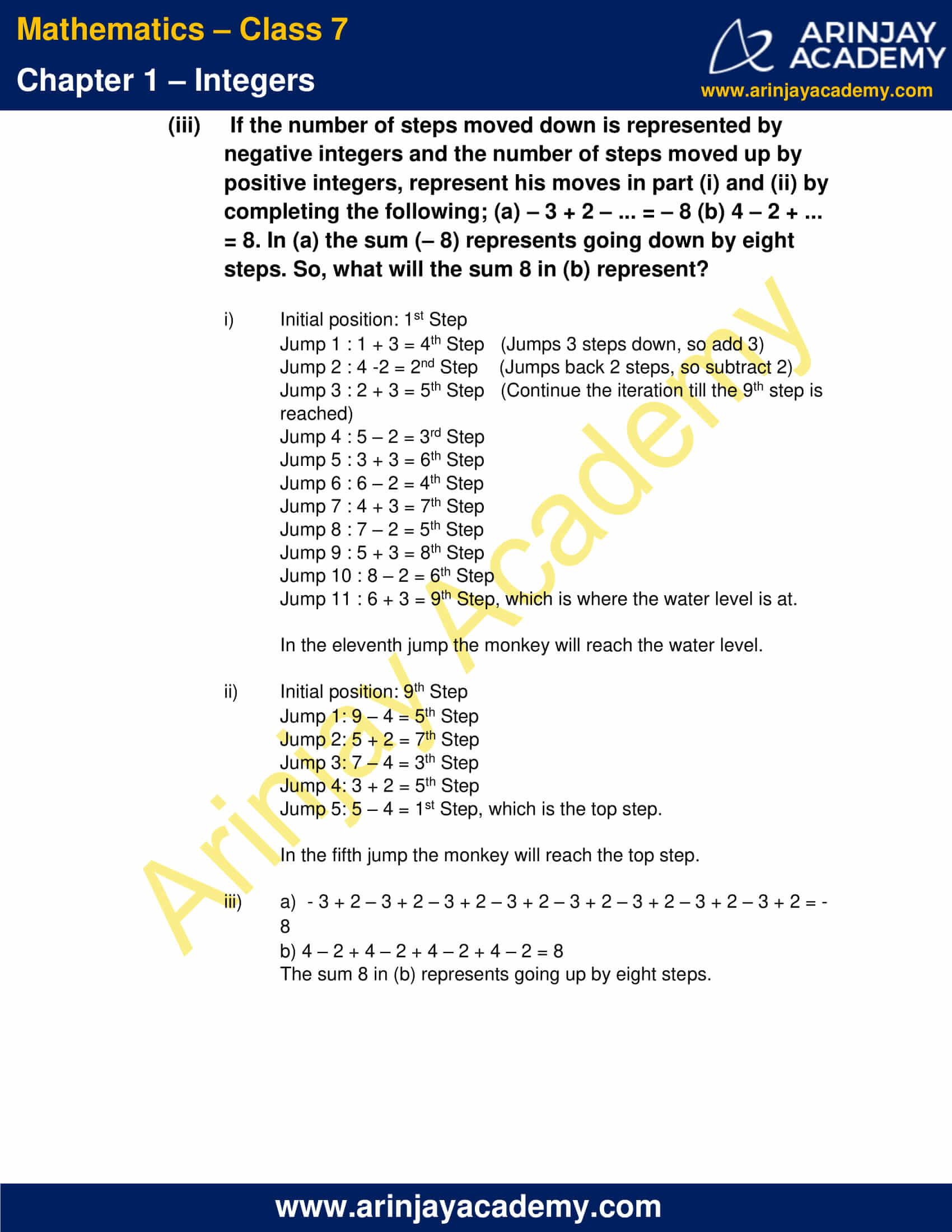### Class 7 Maths NCERT Solutions Chapter 1 – Exercise 1.2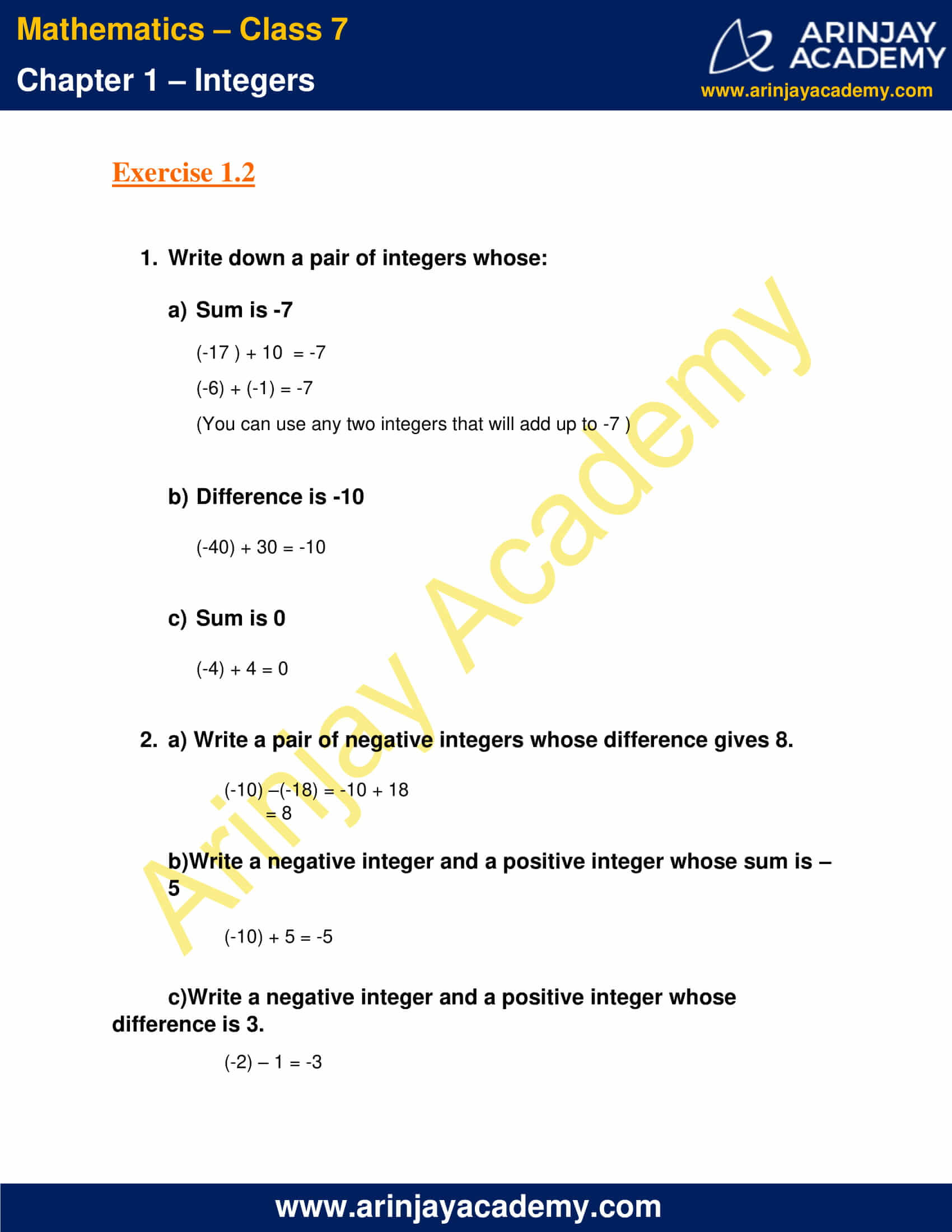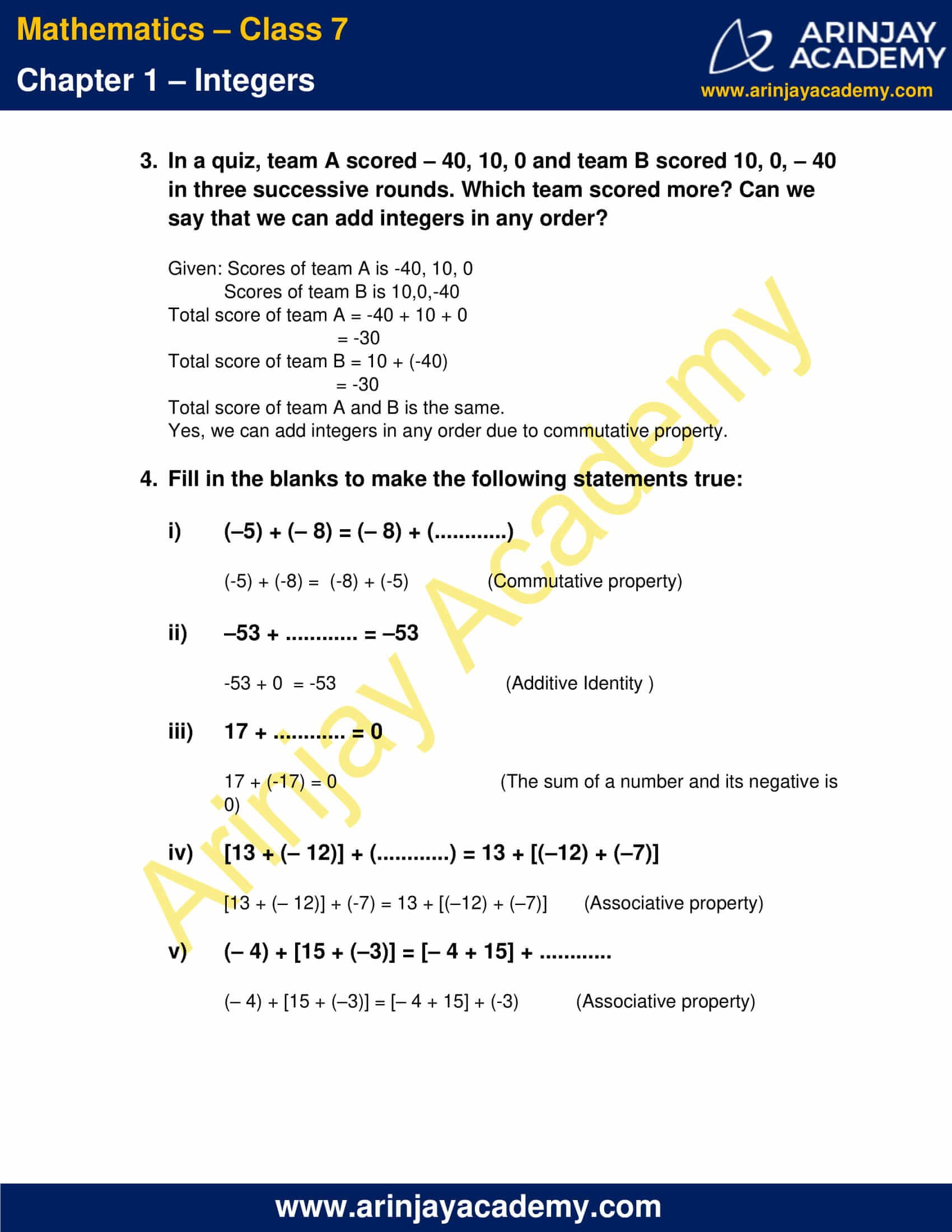### Class 7 Maths NCERT Solutions Chapter 1 – Exercise 1.3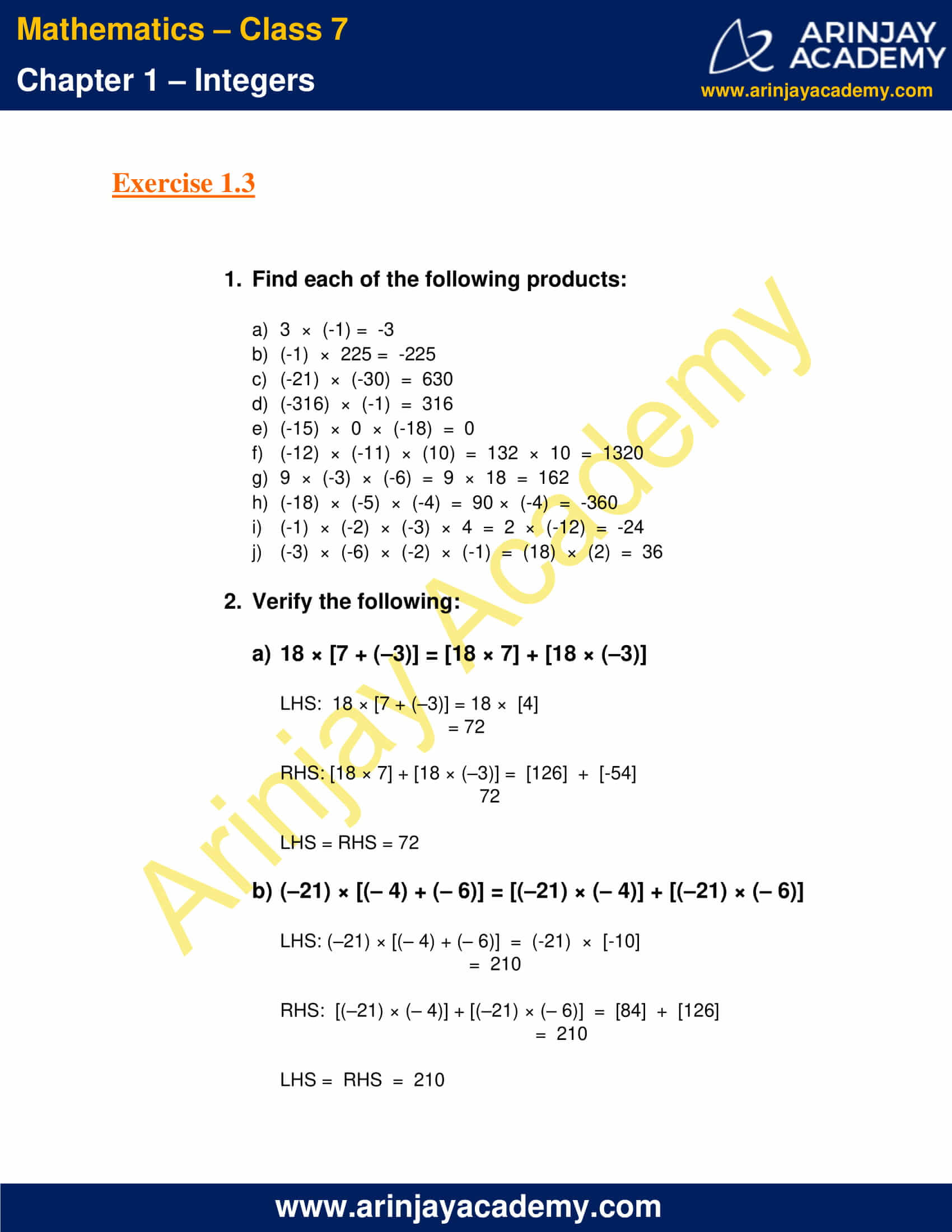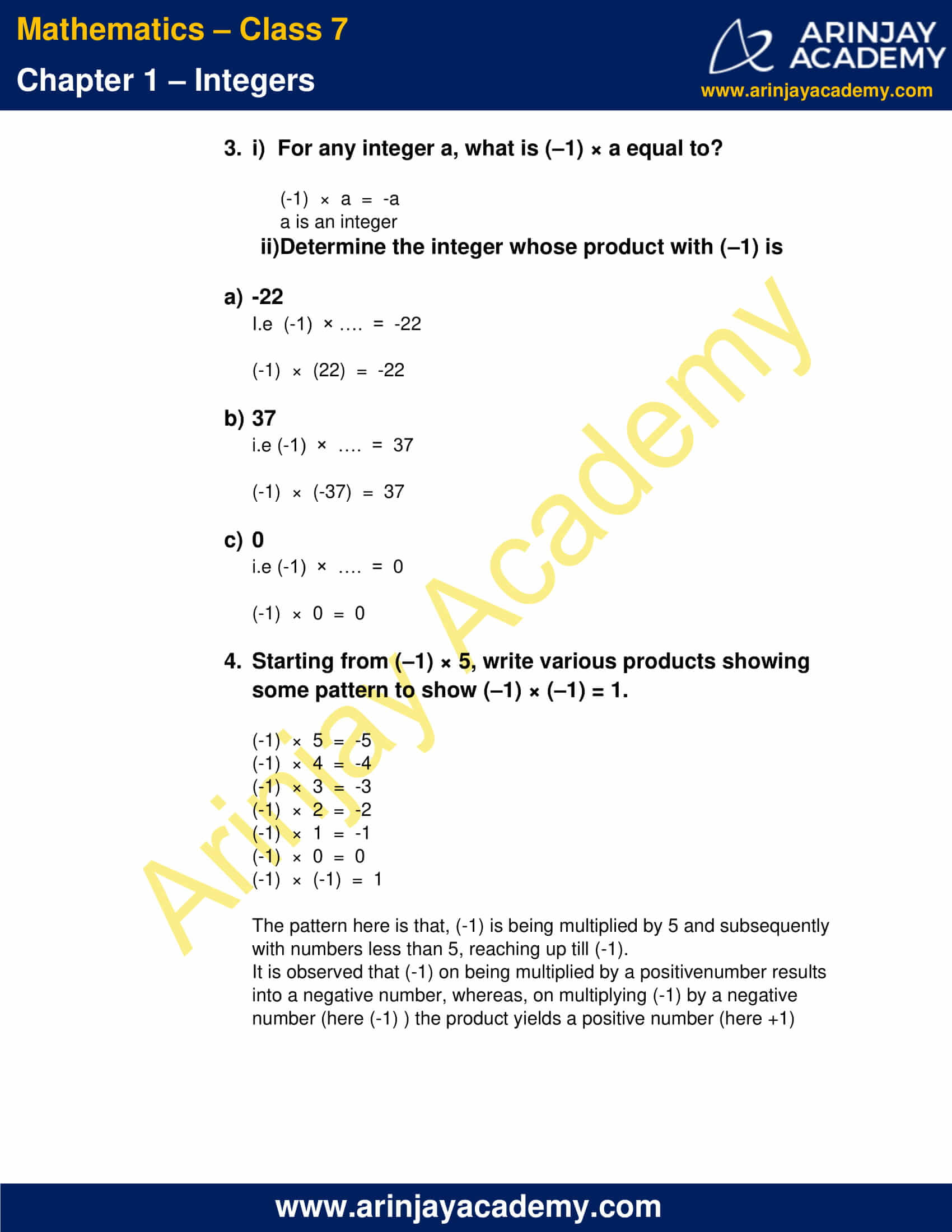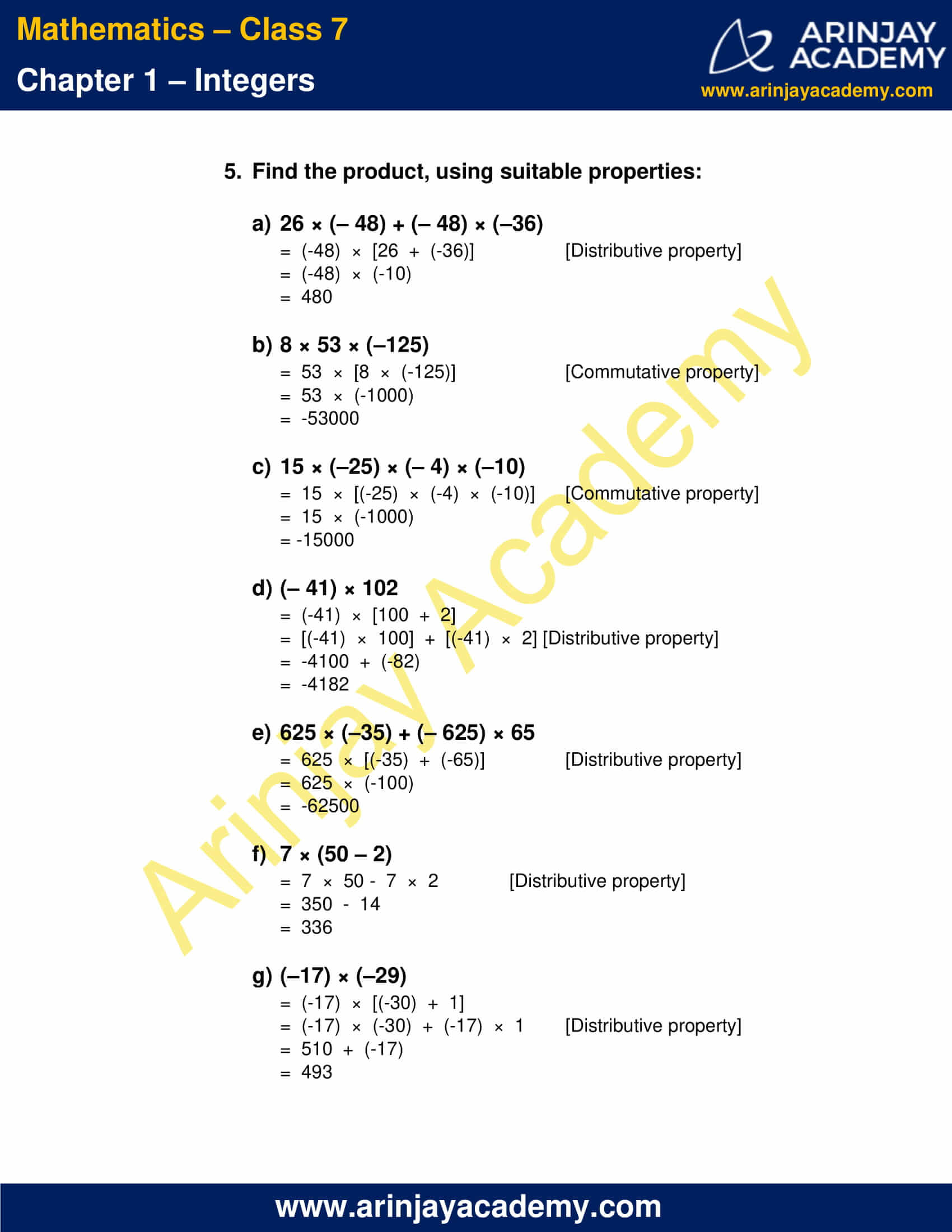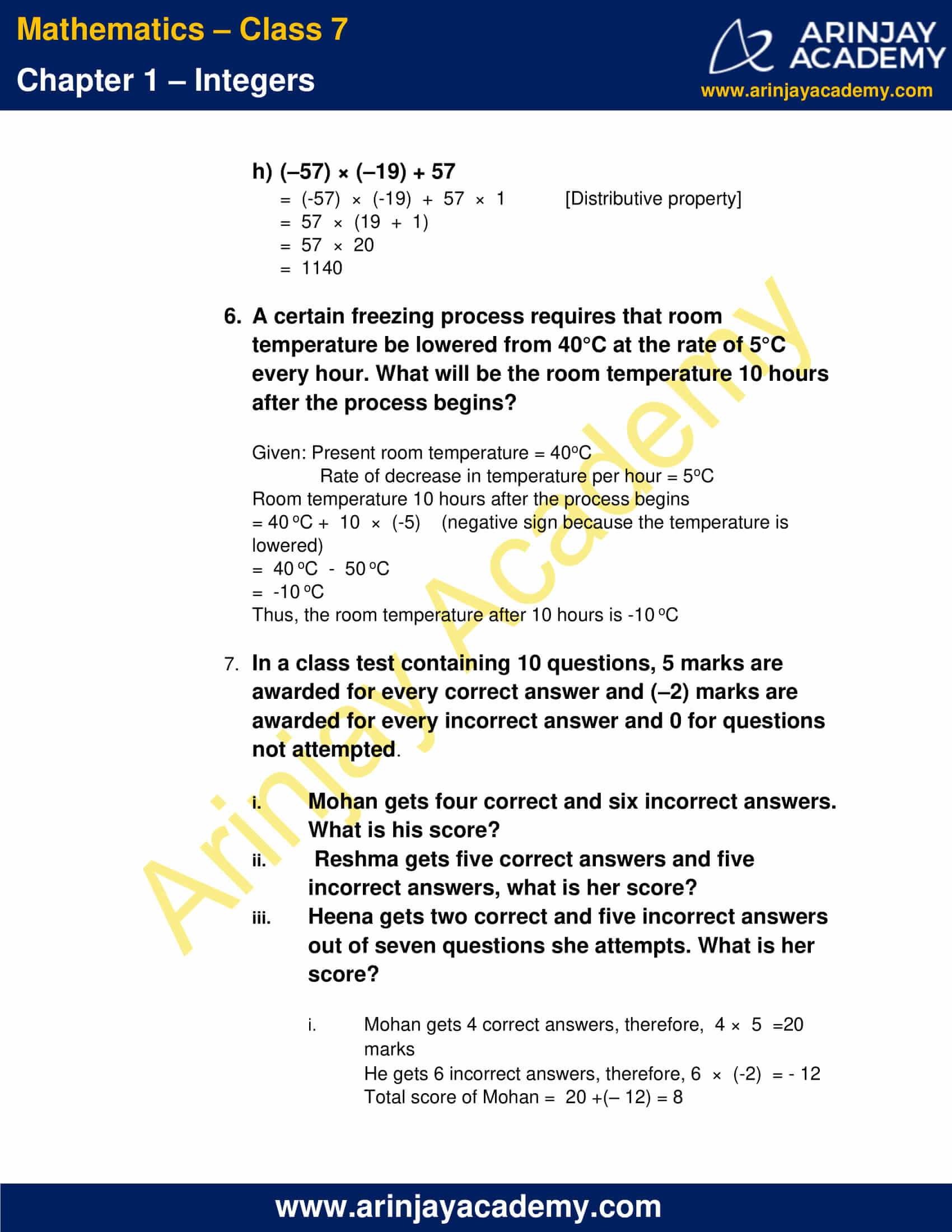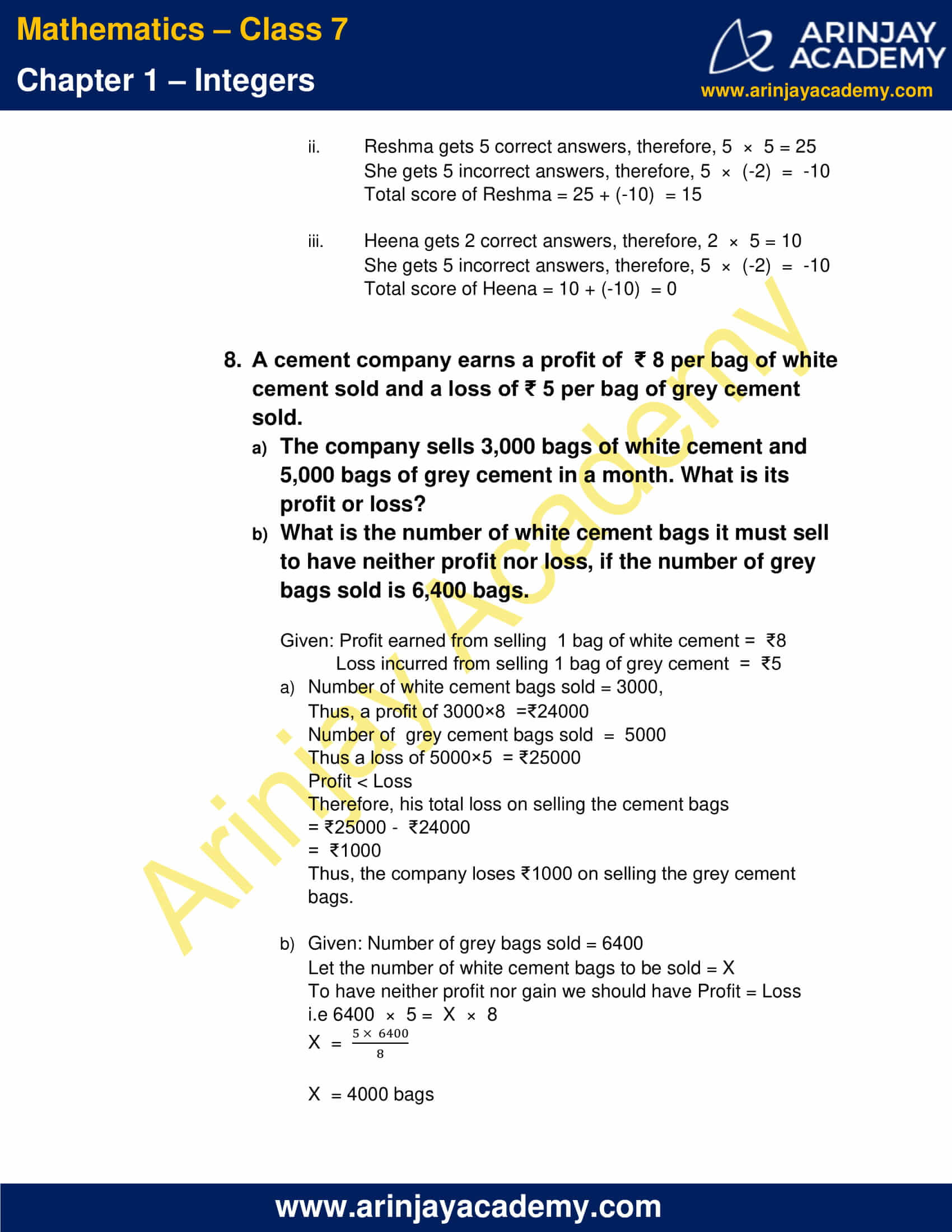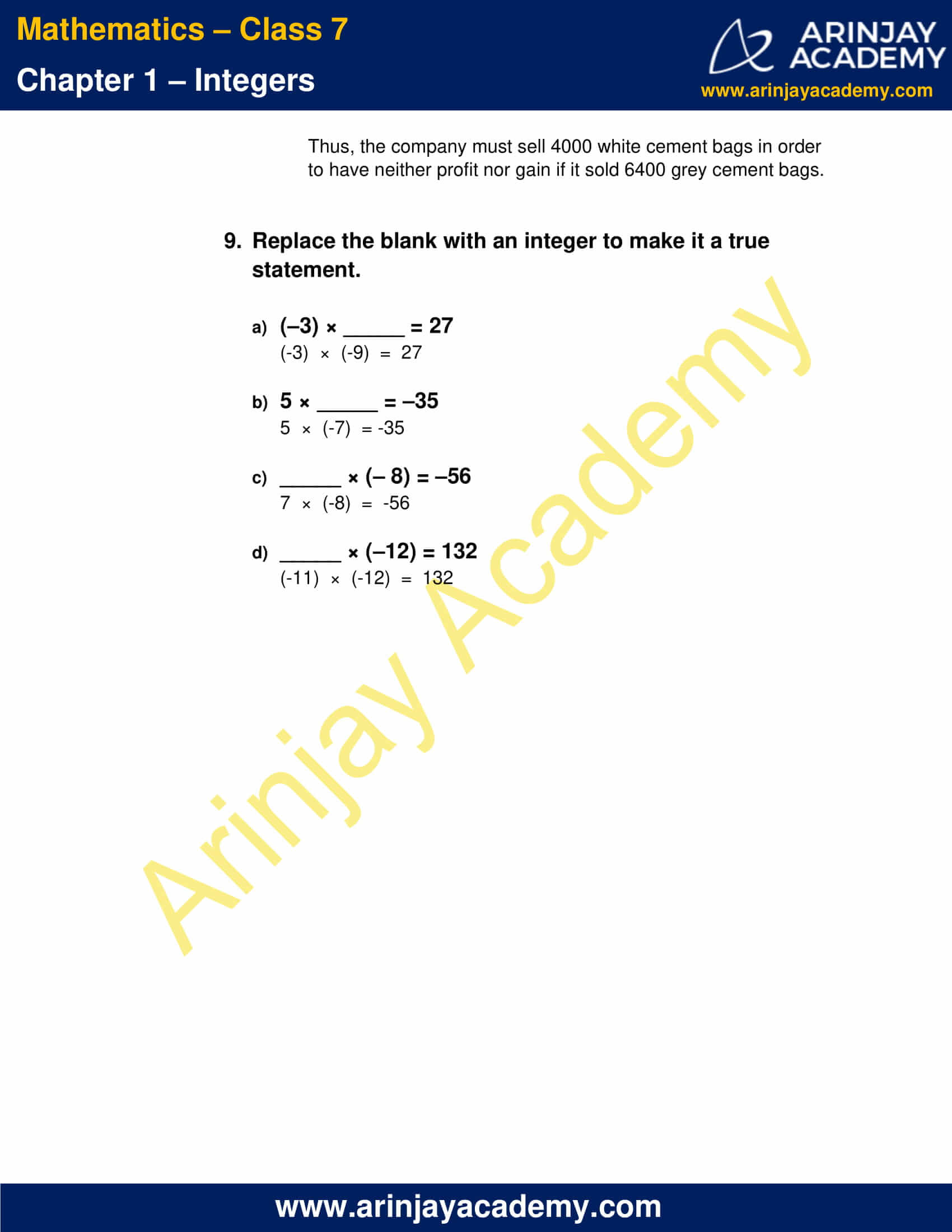### Class 7 Maths NCERT Solutions Chapter 1 – Exercise 1.4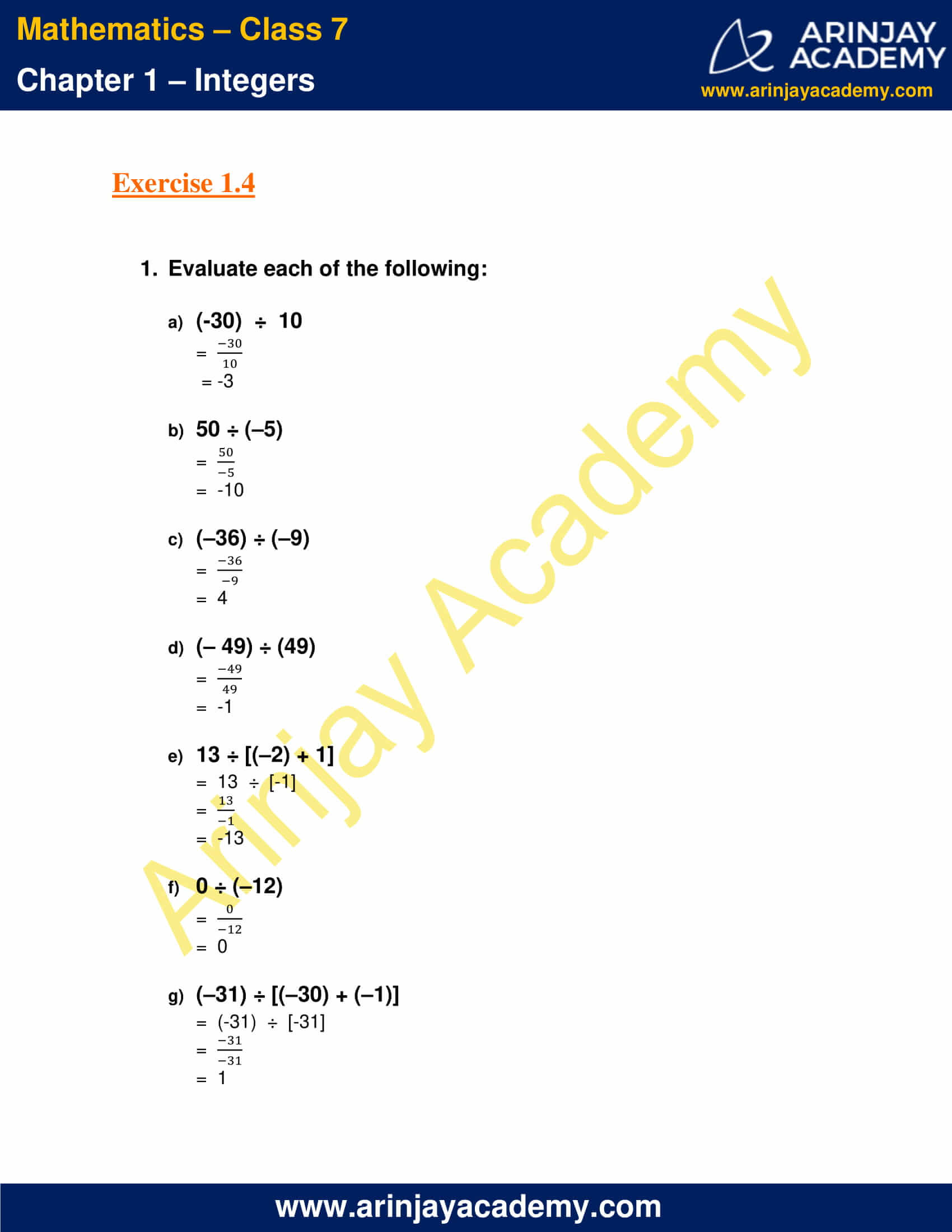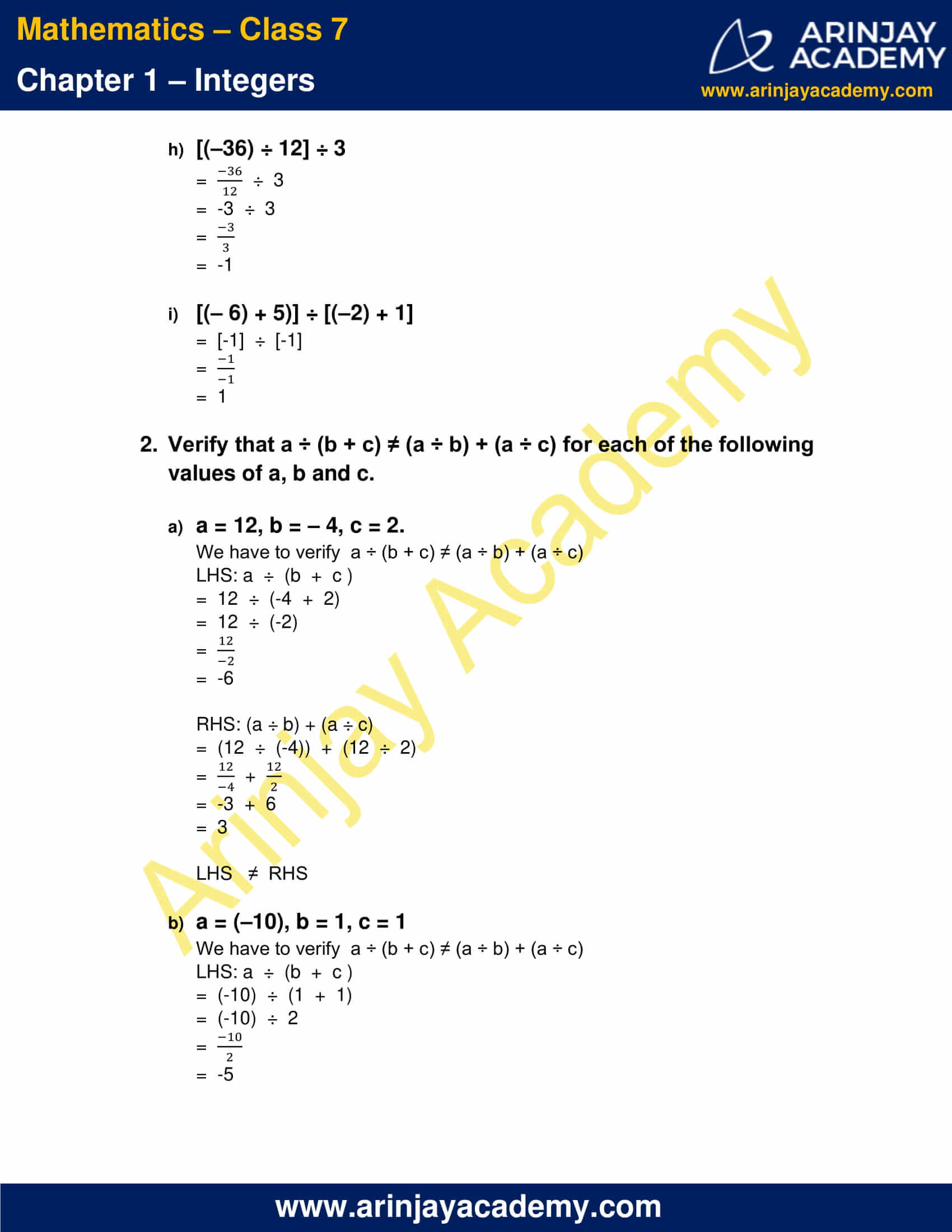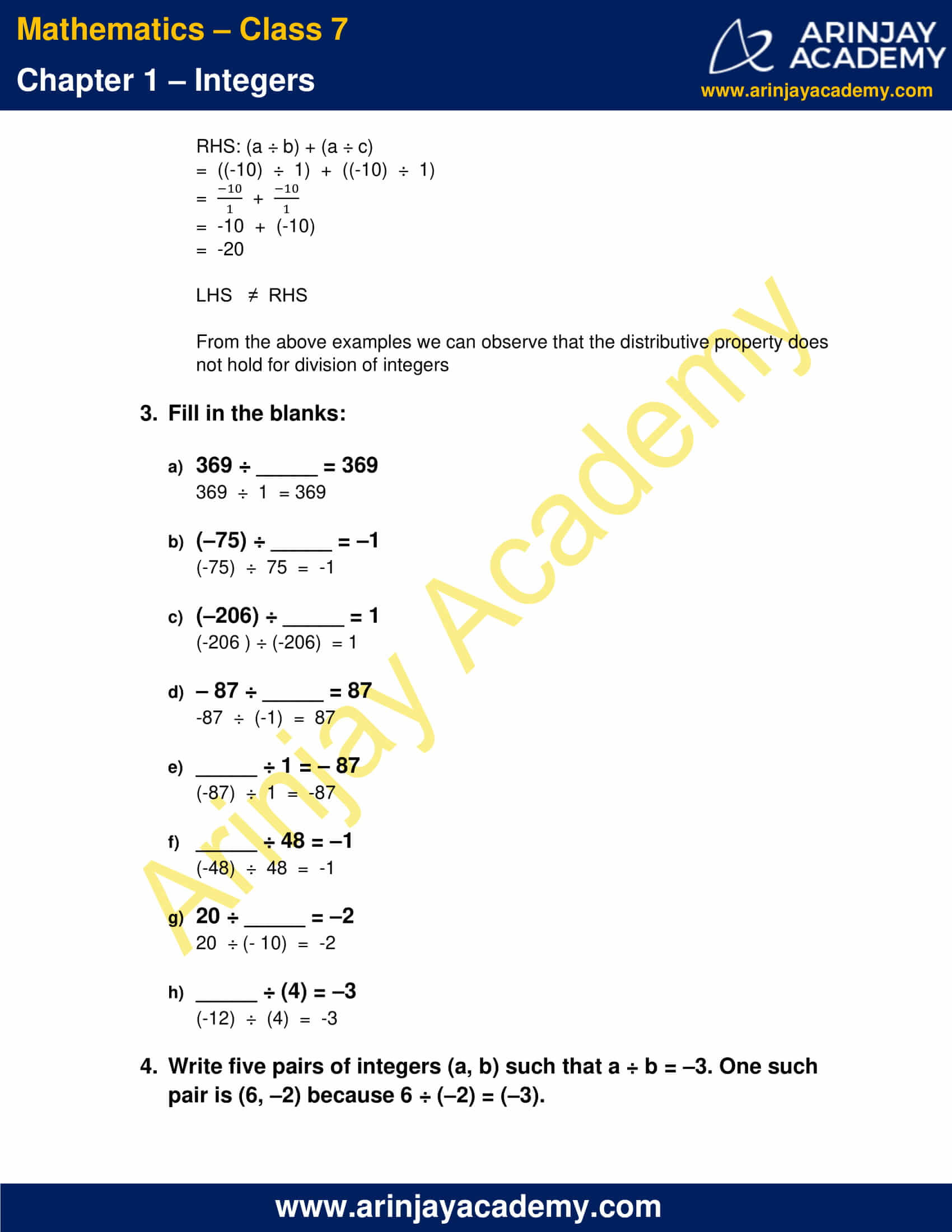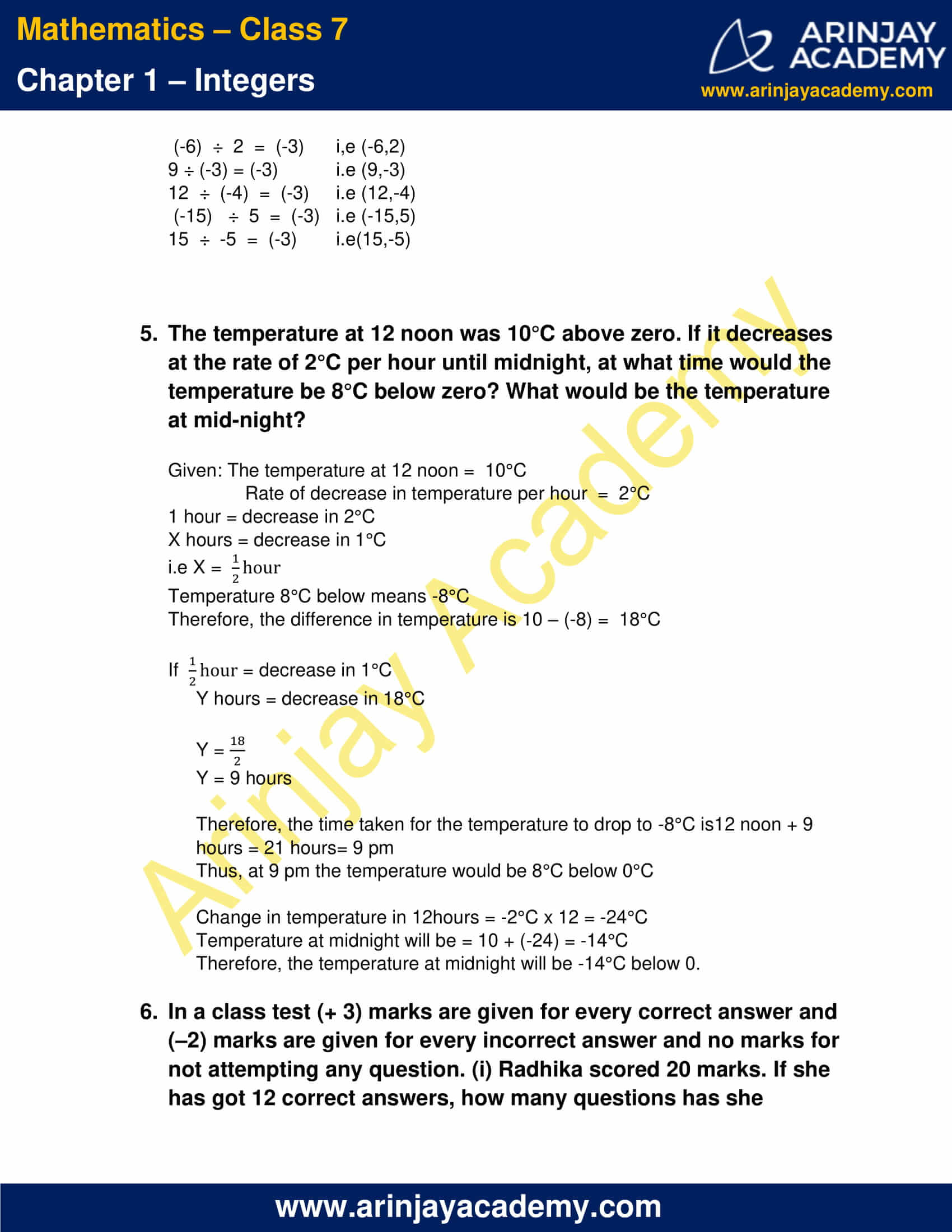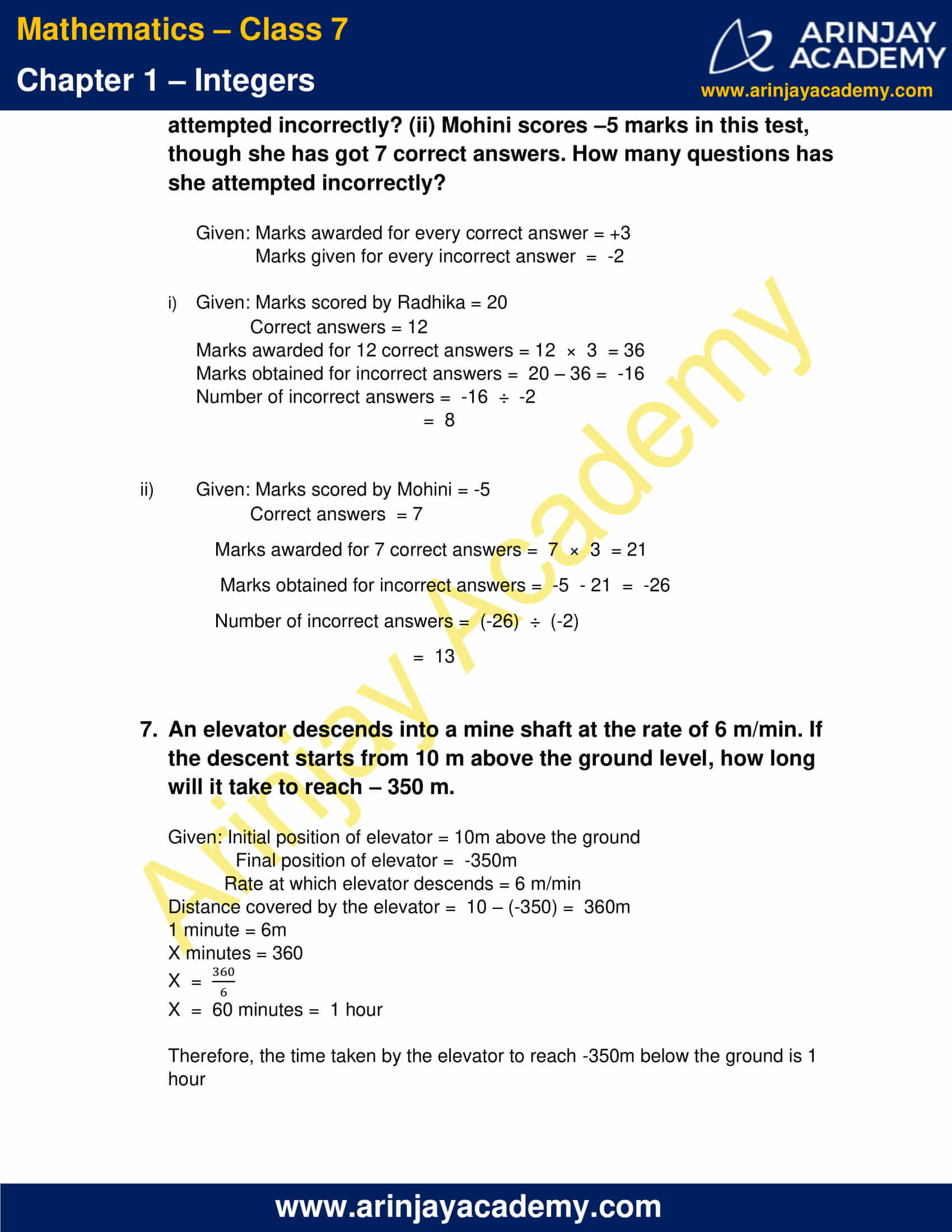### Class 7 Maths NCERT Solutions Chapter 1 – Exercise 1.1

1. Following number line shows the temperature in degree Celsius (°C) at different places on a particular day.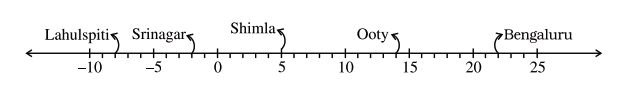(a) Observe this number line and write the temperature of the places marked on it.
(b) What is the temperature difference between the hottest and the coldest places among the above
(c) What is the temperature difference between Lahulspiti and Srinagar?
(d) Can we say temperature of Srinagar and Shimla taken together is less than the temperature at Shimla? Is it also less than the temperature at Srinagar?

(a)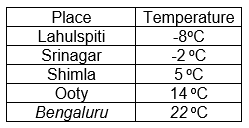(b) Temperature of the hottest place( Bangalore ) = 22 oC
Temperature of the Coldest place (Lahulspiti) = -8 oC
The temperature difference between the hottest and the coldest places = 22 oC – (-8 oC)
= 22 oC + 8 oC
= 30 oC

(c) Temperature of  Srinagar = -2 oC
Temperature of Lahulspiti = -8 oC
The temperature difference between Lahulspiti and Srinagar = -2oC – (-8 oC)
= -2 oC + 8 oC
= 6 oC

(d) Temperature of Srinagar and Shimla taken together is = 5oC +(-2oC)
= 5 oC – 2 oC
= 3 oC
Temperature at Shimla is = 5 oC
Clearly, 3 oC < 5 oC
Therefore, we can say that the temperature of Srinagar and Shimla taken together is less than the temperature at Shimla.
Temperature of Srinagar = -2oC
3 oC > -2oC
Therefore, we can say that the temperature of Srinagar and Shimla taken together is not  less than the temperature at Srinagar.

2. In a quiz, positive marks are given for correct answers and negative marks are given for incorrect answers. If Jack’s scores in five successive rounds were 25, – 5, – 10, 15 and 10, what was his total at the end?

Given: Jack’s score in five successive rounds are 25 , -5 , -10 , 15 and 10
Total marks scored by Jack = 25 + (-5) + (-10) + 15 + 10
= 25 -5 – 10 + 15 + 10
= 25 + 15 + 10 – 5 – 10
= 50 – 15
Total marks scored by Jack = 35

3. At Srinagar temperature was – 5°C on Monday and then it dropped by 2°C on Tuesday. What was the temperature of Srinagar on Tuesday? On Wednesday, it rose by 4°C. What was the temperature on this day?

Given: Temperature on Monday = -5oC
Temperature drop on Tuesday = 2 oC
Temperature rise on Wednesday = 4 oC
Therefore, temperature on Tuesday = -5 oC – 2 oC
= -7 oC
And temperature on Wednesday = -7 oC + 4 oC
= -3 oC
Thus, temperature on Tuesday and Wednesday is -7 oC and -3 oC respectively.

4. A plane is flying at the height of 5000 m above the sea level. At a particular point, it is exactly above a submarine floating 1200 m below the sea level. What is the vertical distance between them?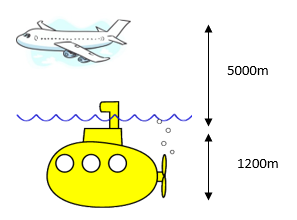Height of the plane above sea level = 5000m
Position of submarine below sea level = 1200m
Vertical distance between the plane and the submarine = 5000 + 1200
Thus, the vertical distance between them =   6200m

5. Mohan deposits ₹2000 in his bank account and withdraws ₹1642 from it, the next day. If withdrawal of amount from the account is represented by a negative integer, then how will you represent the amount deposited? Find the balance in Mohan’s account after the withdrawal.

Given: Amount deposited = ₹2000
Amount withdrawn = ₹1642
If withdrawal of amount from the account is represented by a negative integer, then amount deposited will be represented by a positive number.
Balance = ₹2000 – ₹ 1642 = ₹358
Balance in Mohan’s account after withdrawal is ₹358

6. Rita goes 20 km towards east from a point A to the point B. From B, she moves 30 km towards west along the same road. If the distance towards east is represented by a positive integer then, how will you represent the distance travelled towards west? By which integer will you represent her final position from A?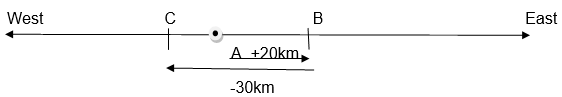If the distance towards east is represented by a positive integer then, distance travelled towards west is represented by a negative integer.
Distance between A and B i.e AB = 20km
Distance between B and C i.e BC = 30km
Distance travelled from A = AB – BC       ( -BC because Rita moves 30km west)
= 20 – 30
= -10km
If A is taken to be the origin, then Rita’s final position from A to C is -10km

7. In a magic square each row, column and diagonal have the same sum. Check which of the following is a magic square.

(i)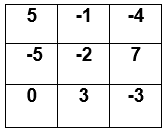[A magic square is a square divided into smaller squares each containing a number, such that the figures in each vertical, horizontal, and diagonal row add up to the same value.]

5 +(-1) + (-4) = 5 – 5 = 0
(-5) + (-2) + 7 = -7 + 7 = 0
0 + 3 + (-3) = 3 – 3 = 0

5 + (-5) + 0 = 5 – 5 = 0
(-1) + (-2) + 3 = -3 + 3 = 0
(-4) + 7 + (-3) = -7 + 7 = 0

5 +(-2) + (-3) = 5 – 5 = 0
(-4) + (-2) + 0 = -4 – 2 = -6

Not a magic square since the sum of one the diagonal rows is not 0

(ii)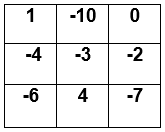1 + (-10) + 0 = 1 – 10 = -9
(-4) + (-3) + (-2) = -4 – 3 – 2  = -9
(-6) + 4 + (-7) = -6 + 4 – 7 = – 9

1 + (-4) + (-6) = 1 – 4 – 6 = -9
(-10) + (-3) + 4 = -10 – 3 + 4 = -9
0 + (-2) +(-7) = -2 – 7 = -9

1 + (-3) + (-7) = 1 – 3 – 7 = -9
0 + (-3) + (-6) = -3 – 6 = -9

It is a magic square since the sum of all the rows is equal; here they add up to -9

8. Verify a – (– b) = a + b for the following values of a and b.

(i) a = 21, b = 18

LHS:  a – (– b) = 21 – (-18)
= 21 + 18
LHS = 39

RHS: a + b = 21 + 18
RHS = 39

LHS = RHS = 39. Hence, verified.

(ii) a = 118, b = 125

LHS:  a – (– b) = 118 – (-125)
= 118 + 125
LHS = 243

RHS: a + b = 118 + 125
RHS = 243

LHS = RHS = 243. Hence, verified.

(iii) a = 75, b = 84

LHS:  a – (– b) = 75 – (-84)
= 75 + 84
LHS = 159

RHS: a + b = 75 + 84
RHS = 159

LHS = RHS = 159. Hence, verified.

(iv) a = 28, b = 11

LHS:  a – (– b) = 28 – (-11)
= 28 –+11
LHS = 39

RHS: a + b = 28 + 11
RHS = 39

LHS = RHS = 39. Hence, verified.

9. Use the sign of >, < or = in the box to make the statements true.

a. (– 8) + (– 4)  <  (–8) – (– 4)

Because,
LHS:   -8 – 4 = -12
RHS:   -8 + 4 = -4

b. (– 3) + 7 – (19) <  15 – 8 + (– 9)

Because,
LHS:   -3 + 7 – 19 =-15
RHS:   15 – 8 – 9 = -2

c. 23 – 41 + 11  >  23 – 41 – 11

Because,
LHS:   23 – 41 + 11 = -7
RHS:   23 – 41 – 11 = -29

d. 39 + (– 24) – (15) < 36 + (– 52) – (– 36)

Because,
LHS:   39 – 24 – 15 = 0
RHS:   36 – 52 + 36 = 20

e. – 231 + 79 + 51  >  –399 + 159 + 81

Because,
LHS:    -231 + 79 + 51 = -101
RHS:    -399 + 159 + 81 = -159

10. A water tank has steps inside it. A monkey is sitting on the topmost step (i.e., the first step). The water level is at the ninth step.

(i) He jumps 3 steps down and then jumps back 2 steps up. In how many jumps will he reach the water level?

(ii) After drinking water, he wants to go back. For this, he jumps 4 steps up and then jumps back 2 steps down in every move. In how many jumps will he reach back the top step?

(iii) If the number of steps moved down is represented by negative integers and the number of steps moved up by positive integers, represent his moves in part (i) and (ii) by completing the following; (a) – 3 + 2 – … = – 8 (b) 4 – 2 + … = 8. In (a) the sum (– 8) represents going down by eight steps. So, what will the sum 8 in (b) represent?

(i) Initial position: 1st Step
Jump 1 : 1 + 3 = 4th Step   (Jumps 3 steps down, so add 3)
Jump 2 : 4 -2 = 2nd Step    (Jumps back 2 steps, so subtract 2)
Jump 3 : 2 + 3 = 5th Step   (Continue the iteration till the 9th step is reached)
Jump 4 : 5 – 2 = 3rd Step
Jump 5 : 3 + 3 = 6th Step
Jump 6 : 6 – 2 = 4th Step
Jump 7 : 4 + 3 = 7th Step
Jump 8 : 7 – 2 = 5th Step
Jump 9 : 5 + 3 = 8th Step
Jump 10 : 8 – 2 = 6th Step
Jump 11 : 6 + 3 = 9th Step, which is where the water level is at.
In the eleventh jump the monkey will reach the water level.

(ii) Initial position: 9th Step
Jump 1: 9 – 4 = 5th Step
Jump 2: 5 + 2 = 7th Step
Jump 3: 7 – 4 = 3th Step
Jump 4: 3 + 2 = 5th Step
Jump 5: 5 – 4 = 1st Step, which is the top step.
In the fifth jump the monkey will reach the top step.

(iii) a) – 3 + 2 – 3 + 2 – 3 + 2 – 3 + 2 – 3 + 2 – 3 + 2 – 3 + 2 – 3 + 2 = – 8
b) 4 – 2 + 4 – 2 + 4 – 2 + 4 – 2 = 8
The sum 8 in (b) represents going up by eight steps.

### Class 7 Maths NCERT Solutions Chapter 1 – Exercise 1.2

1. Write down a pair of integers whose:

a) Sum is -7
(-17 ) + 10  = -7
(-6) + (-1) = -7
(You can use any two integers that will add up to -7 )

b) Difference is -10
(-40) + 30 = -10

c) Sum is 0
(-4) + 4 = 0

2. a) Write a pair of negative integers whose difference gives 8.
(-10) –(-18) = -10 + 18
= 8

b) Write a negative integer and a positive integer whose sum is –5
(-10) + 5 = -5

c) Write a negative integer and a positive integer whose difference is 3.
(-2) – 1 = -3

3. In a quiz, team A scored – 40, 10, 0 and team B scored 10, 0, – 40 in three successive rounds. Which team scored more? Can we say that we can add integers in any order?

Given: Scores of team A is -40, 10, 0
Scores of team B is 10,0,-40
Total score of team A = -40 + 10 + 0
= -30
Total score of team B = 10 + (-40)
= -30
Total score of team A and B is the same.
Yes, we can add integers in any order due to commutative property.

4. Fill in the blanks to make the following statements true:

i) (–5) + (– 8) = (– 8) + (…………)
(-5) + (-8) =  (-8) + (-5)  (Commutative property)

ii) –53 + ………… = –53
-53 + 0  = -53  (Additive Identity )

iii) 17 + ………… = 0
17 + (-17) = 0   (The sum of a number and its negative is 0)

iv) [13 + (– 12)] + (…………) = 13 + [(–12) + (–7)]
[13 + (– 12)] + (-7) = 13 + [(–12) + (–7)]  (Associative property)

v) (– 4) + [15 + (–3)] = [– 4 + 15] + …………
(– 4) + [15 + (–3)] = [– 4 + 15] + (-3)  (Associative property)

### Class 7 Maths NCERT Solutions Chapter 1 – Exercise 1.3

1. Find each of the following products:

a) 3 ×  (-1) =  -3
b) (-1) ×  225 =  -225
c) (-21) ×  (-30)  =  630
d) (-316) ×  (-1)  =  316
e) (-15) ×  0  ×  (-18)  =  0
f) (-12) ×  (-11)  ×  (10)  =  132  ×  10  =  1320
g) 9 ×  (-3)  ×  (-6)  =  9  ×  18  =  162
h) (-18) ×  (-5)  ×  (-4)  =  90 ×  (-4)  =  -360
i) (-1) ×  (-2)  ×  (-3)  ×  4  =  2  ×  (-12)  =  -24
j) (-3) ×  (-6)  ×  (-2)  ×  (-1)  =  (18)  ×  (2)  =  36

2. Verify the following:

a) 18 × [7 + (–3)] = [18 × 7] + [18 × (–3)]

LHS:  18 × [7 + (–3)] = 18 ×  
= 72

RHS: [18 × 7] + [18 × (–3)] =    +  [-54]
= 72

LHS = RHS = 72

b) (–21) × [(– 4) + (– 6)] = [(–21) × (– 4)] + [(–21) × (– 6)]

LHS: (–21) × [(– 4) + (– 6)]  =  (-21)  ×  [-10]
=  210

RHS:  [(–21) × (– 4)] + [(–21) × (– 6)]  =    +  
=  210

LHS =  RHS  =  210

3. i)  For any integer a, what is (–1) × a equal to?

(-1)  ×  a  =  -a
a is an integer

ii) Determine the integer whose product with (–1) is

a) -22

i.e  (-1)  × ….  =  -22
(-1)  ×  (22)  =  -22

b) 37

i.e (-1)  ×  ….  =  37
(-1)  ×  (-37)  =  37

c) 0

i.e (-1)  ×  ….  =  0
(-1)  ×  0  =  0

4. Starting from (–1) × 5, write various products showing some pattern to show (–1) × (–1) = 1.

(-1)  ×  5  =  -5
(-1)  ×  4  =  -4
(-1)  ×  3  =  -3
(-1)  ×  2  =  -2
(-1)  ×  1  =  -1
(-1)  ×  0  =  0
(-1)  ×  (-1)  =  1

The pattern here is that, (-1) is being multiplied by 5 and subsequently with numbers less than 5, reaching up till (-1). It is observed that (-1) on being multiplied by a positive number results into a negative number, whereas, on multiplying (-1) by a negative number (here (-1) ) the product yields a positive number (here +1)

5. Find the product, using suitable properties:

a) 26 × (– 48) + (– 48) × (–36)
=  (-48)  ×  [26  +  (-36)]    [Distributive property]
=  (-48)  ×  (-10)
=  480

b) 8 × 53 × (–125)
=  53  ×  [8  ×  (-125)]  [Commutative property]
=  53  ×  (-1000)
=  -53000

c) 15 × (–25) × (– 4) × (–10)
=  15  ×  [(-25)  ×  (-4)  ×  (-10)] [Commutative property]
=  15  ×  (-1000)
= -15000

d) (– 41) × 102
=  (-41)  ×  [100  +  2]
=  [(-41)  ×  100]  +  [(-41)  ×  2] [Distributive property]
=  -4100  +  (-82)
=  -4182

e) 625 × (–35) + (– 625) × 65
=  625  ×  [(-35)  +  (-65)]  [Distributive property]
=  625  ×  (-100)
=  -62500

f) 7 × (50 – 2)
=  7  ×  50 –  7  ×  2   [Distributive property]
=  350  –  14
=  336

g) (–17) × (–29)
=  (-17)  ×  [(-30)  +  1]
=  (-17)  ×  (-30)  +  (-17)  ×  1   [Distributive property]
=  510  +  (-17)
=  493

h) (–57) × (–19) + 57
=  (-57)  ×  (-19)  +  57  ×  1  [Distributive property]
=  57  ×  (19  +  1)
=  57  ×  20
=  1140

6. A certain freezing process requires that room temperature be lowered from 40°C at the rate of 5°C every hour. What will be the room temperature 10 hours after the process begins?

Given: Present room temperature = 40oC
Rate of decrease in temperature per hour = 5oC
Room temperature 10 hours after the process begins
= 40 oC +  10  ×  (-5)    (negative sign because the temperature is lowered)
=  40 oC  –  50 oC
=  -10 oC
Thus, the room temperature after 10 hours is -10 oC

7. In a class test containing 10 questions, 5 marks are awarded for every correct answer and (–2) marks are awarded for every incorrect answer and 0 for questions not attempted.

(i) Mohan gets four correct and six incorrect answers. What is his score?
(ii) Reshma gets five correct answers and five incorrect answers, what is her score?
(iii) Heena gets two correct and five incorrect answers out of seven questions she attempts. What is her score?

(i) Mohan gets 4 correct answers, therefore, 4 ×  5  =20 marks
He gets 6 incorrect answers, therefore, 6  ×  (-2)  = – 12
Total score of Mohan =  20 +(– 12) = 8

(ii) Reshma gets 5 correct answers, therefore, 5 ×  5 = 25
She gets 5 incorrect answers, therefore, 5  ×  (-2)  =  -10
Total score of Reshma = 25 + (-10)  = 15

(iii) Heena gets 2 correct answers, therefore, 2 ×  5 = 10
She gets 5 incorrect answers, therefore, 5  ×  (-2)  =  -10
Total score of Heena = 10 + (-10)  = 0

8. A cement company earns a profit of ₹ 8 per bag of white cement sold and a loss of ₹ 5 per bag of grey cement sold.

a) The company sells 3,000 bags of white cement and 5,000 bags of grey cement in a month. What is its profit or loss?

b) What is the number of white cement bags it must sell to have neither profit nor loss, if the number of grey bags sold is 6,400 bags.

Given: Profit earned from selling  1 bag of white cement =  ₹8
Loss incurred from selling 1 bag of grey cement  =  ₹5

a) Number of white cement bags sold = 3000,
Thus, a profit of 3000×8  =₹24000
Number of  grey cement bags sold  =  5000
Thus a loss of 5000×5  = ₹25000
Profit < Loss
Therefore, his total loss on selling the cement bags = ₹25000 –  ₹24000
=  ₹1000
Thus, the company loses ₹1000 on selling the grey cement bags.

b) Given: Number of grey bags sold = 6400
Let the number of white cement bags to be sold = X
To have neither profit nor gain we should have Profit = Loss
i.e 6400  ×  5 =  X  ×  8
X  = (5 x 6400)/8
X  = 4000 bags
Thus, the company must sell 4000 white cement bags in order to have neither profit nor gain if it sold 6400 grey cement bags.

9. Replace the blank with an integer to make it a true statement.

a) (–3) × _____ = 27
(-3)  ×  (-9)  =  27

b) 5 × _____ = –35
5  ×  (-7)  = -35

c) _____ × (– 8) = –56
7  ×  (-8)  =  -56

d) _____ × (–12) = 132
(-11)  ×  (-12)  =  132

### Class 7 Maths NCERT Solutions Chapter 1 – Exercise 1.4

1. Evaluate each of the following:

a) (-30) ÷  10
=  -30/10
= -3

b) 50 ÷ (–5)

=  50/-5
=  -10

c) (–36) ÷ (–9)

= -36/-9
=  4

d) (– 49) ÷ (49)
= -49/49
=  -1

e) 13 ÷ [(–2) + 1]
=  13  ÷  [-1]
= 13/-1
=  -13

f) 0 ÷ (–12)
= 0/(-12)
=  0

g) (–31) ÷ [(–30) + (–1)]
=  (-31)  ÷  [-31]
= -31/-31
=  1

h) [(–36) ÷ 12] ÷ 3
=  -36/12 ÷  3
=  -3  ÷  3
= -3/3
=  -1

i) [(– 6) + 5)] ÷ [(–2) + 1]
=  [-1]  ÷  [-1]
= -1/-1
=  1

2. Verify that a ÷ (b + c) ≠ (a ÷ b) + (a ÷ c) for each of the following values of a, b and c.

(a) a = 12, b = – 4, c = 2.

We have to verify  a ÷ (b + c) ≠ (a ÷ b) + (a ÷ c)

LHS: a  ÷  (b  +  c )
=  12  ÷  (-4  +  2)
=  12  ÷  (-2)
= 12/-2
=  -6

RHS: (a ÷ b) + (a ÷ c)
=  (12  ÷  (-4))  +  (12  ÷  2)
=  12/-4 + 12/2
=  -3  +  6
=  3

LHS   ≠  RHS

(b) a = (–10), b = 1, c = 1

We have to verify  a ÷ (b + c) ≠ (a ÷ b) + (a ÷ c)

LHS: a  ÷  (b  +  c )
=  (-10)  ÷  (1  +  1)
=  (-10)  ÷  2
= -10/2
=  -5

RHS: (a ÷ b) + (a ÷ c)
=  ((-10)  ÷  1)  +  ((-10)  ÷  1)
=  -10/1  + -10/1
=  -10  +  (-10)
=  -20

LHS   ≠  RHS

From the above examples we can observe that the distributive property does not hold for division of integers

3. Fill in the blanks:

a) 369 ÷ _____ = 369
369  ÷  1  = 369

b) (–75) ÷ _____ = –1
(-75)  ÷  75  =  -1

c) (–206) ÷ _____ = 1
(-206 ) ÷ (-206)  = 1

d) – 87 ÷ _____ = 87
-87  ÷  (-1)  =  87

e) _____ ÷ 1 = – 87
(-87)  ÷  1  =  -87

f) _____ ÷ 48 = –1
(-48)  ÷  48  =  -1

g) 20 ÷ _____ = –2
20  ÷ (- 10)  =  -2

h) _____ ÷ (4) = –3
(-12)  ÷  (4)  =  -3

4. Write five pairs of integers (a, b) such that a ÷ b = –3. One such pair is (6, –2) because 6 ÷ (–2) = (–3).

(-6)  ÷  2  =  (-3)  i,e (-6,2)
9 ÷ (-3) = (-3)  i.e (9,-3)
12  ÷  (-4)  =  (-3)  i.e (12,-4)
(-15)   ÷  5  =  (-3)  i.e (-15,5)
15  ÷  -5  =  (-3)  i.e(15,-5)

5. The temperature at 12 noon was 10°C above zero. If it decreases at the rate of 2°C per hour until midnight, at what time would the temperature be 8°C below zero? What would be the temperature at mid-night?

Given: The temperature at 12 noon =  10°C
Rate of decrease in temperature per hour  =  2°C
1 hour = decrease in 2°C
X hours = decrease in 1°C
i.e X = 1/2 hour
Temperature 8°C below means -8°C
Therefore, the difference in temperature is 10 – (-8) =  18°C
If   = decrease in 1°C
Y hours = decrease in 18°C
Y = 18/2 hours
Y = 9 hours
Therefore, the time taken for the temperature to drop to -8°C is 12 noon + 9 hours = 21 hours = 9 pm
Thus, at 9 pm the temperature would be 8°C below 0°C

Change in temperature in 12 hours = -2°C x 12 = -24°C
Temperature at midnight will be = 10 + (-24) = -14°C
Therefore, the temperature at midnight will be -14°C below 0.

6. In a class test (+ 3) marks are given for every correct answer and (–2) marks are given for every incorrect answer and no marks for not attempting any question. (i) Radhika scored 20 marks. If she has got 12 correct answers, how many questions has she attempted incorrectly? (ii) Mohini scores –5 marks in this test, though she has got 7 correct answers. How many questions has she attempted incorrectly?

Given: Marks awarded for every correct answer = +3
Marks given for every incorrect answer  =  -2

i) Given: Marks scored by Radhika = 20
Marks awarded for 12 correct answers = 12  ×  3  = 36
Marks obtained for incorrect answers =  20 – 36 =  -16
Number of incorrect answers =  -16  ÷  -2 =  8

ii) Given: Marks scored by Mohini = -5
Marks awarded for 7 correct answers =  7  ×  3  = 21
Marks obtained for incorrect answers =  -5  – 21  =  -26
Number of incorrect answers =  (-26)  ÷  (-2) =  13

7. An elevator descends into a mine shaft at the rate of 6 m/min. If the descent starts from 10 m above the ground level, how long will it take to reach – 350 m.

Given: Initial position of elevator = 10m above the ground
Final position of elevator =  -350m
Rate at which elevator descends = 6 m/min
Distance covered by the elevator =  10 – (-350) =  360m
1 minute = 6m
X minutes = 360
X  = 360/6
X  =  60 minutes =  1 hour
Therefore, the time taken by the elevator to reach -350m below the ground is 1 hour

With this we come to the end of NCERT Solutions for Class 7 Maths Chapter 1 Integers. We hope these helped you study your subject.